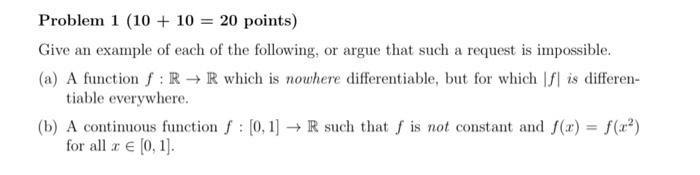# (Solved): Problem $$1(10+10=20$$ points $$)$$ Give an example of each of the following, or argue that suc ...Problem $$1(10+10=20$$ points $$)$$ Give an example of each of the following, or argue that such a request is impossible. (a) A function $$f: \mathbb{R} \rightarrow \mathbb{R}$$ which is nowhere differentiable, but for which $$|f|$$ is differentiable everywhere. (b) A continuous function $$f:[0,1] \rightarrow \mathbb{R}$$ such that $$f$$ is not constant and $$f(x)=f\left(x^{2}\right)$$ for all $$x \in[0,1]$$.

We have an Answer from Expert